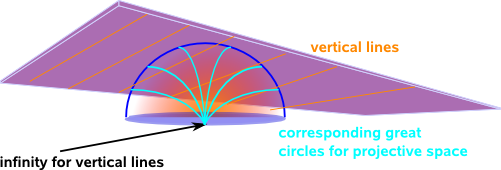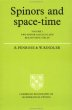# Maths - Hemisphere 2D Derivation

Here we look at a two dimensional euclidean space embedded in a three dimensional projective space, we are using the hemisphere model to do this projection.In two dimensional projective space using hemisphere model:

• Straight lines in euclidean space map to great circles (or semi circles) in projective space.
• Horizontal lines meet at (±1,0,0) which is the point at infinity for horizontal lines.
• Vertical lines meet at (0,±1,0) which is the point at infinity for vertical lines.
• The line at infinity is the line around the sphere at z=0, a point on this line represents direction.

From the above we can see that, as we move away from the origin, lines that cross at 90° in euclidean space do not cross at 90° in projective space. So angles away from the origin are not conserved by the projection.## Derivation for translation between projective (hemisphere) and euclidean spaces

Since we are projecting using a straight line then there is a linear relationship between a point on the plane and its projection on the sphere:

 x y z
= λ
 u v 1

where:

• (x,y,z) = projective coordinates
• (u,v) = euclidean coordinates
• λ = expansion factor of vector: function of (x,y,z) or (u,v)

Dividing the top two rows by the bottom row gives the euclidean coodinates in terms of the projective coordinates:

 u v
=
 x/z y/z

To go the other way from the projective coordinates to the euclidean coodinates we need to use the information that the coordinates are constrained to a unit sphere so:

x² + y² + z² = 1

rearanging gives:

x²/z² + y²/z² + 1 = 1/z²

u² + v² + 1 = 1/λ²

λ = ±1/√(u² + v² + 1)

substituting this into the first vector equation gives:

 x y z
= ±1/√(u² + v² + 1)
 u v 1Roger Penrose - The Road to Reality: Partly a 'popular science' book as it tries to minimise the number of equations (Not that I'm complaining much his book 'Spinors and space-time' went over my head in the first few pages) it still has lots of interesting results that its difficult to find elsewhere.Spinors and Space-Time: Volume 1, Two-Spinor Calculus and Relativistic Fields (Cambridge Monographs on Mathematical Physics) by Roger Penrose and Wolfgang Rindler - This book is about the mathematics of special relativity, it very quickly goes over my head by I hope I will understand it one day.